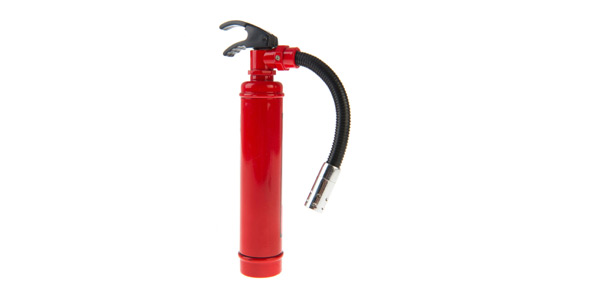# Let's Check Your Knowledge Of Properties Of Gas...

7 Questions | Total Attempts: 144SettingsTake this test to test your knowledge of the topic - properties of gas. If your score is not upto the mark then work hard and prepare the topic well.

• 1.
The perfect example of an ideal gas is
• A.

Air

• B.

Hydrogen

• C.

Water vapor

• D.

None of the above

• 2.
What is an ideal gas?
• A.

Which obeys the law pv = RT

• B.

Which obeys the law p = vR / T

• C.

Which obeys the law pv = R / T

• D.

None of the above

• 3.
According to the Avogadro's law, what is the relation between volume of 1 kg mol of oxygen and volume of 1 kg mol of nitrogen, at normal pressure and temperature? (Mass of 1 kg mol of oxygen is 32 kg and mass of 1 kg mol of nitrogen is 28 kg)
• A.

Volume of 1 kg mol of oxygen is greater than that of nitrogen

• B.

Volume of 1 kg mol of oxygen is less than that of nitrogen

• C.

Volume of 1 kg mol of oxygen is same as that of nitrogen

• D.

None of the above

• 4.
In which condition can real gas closely obey the ideal gas equation?
• A.

Pressure is very small and temperature is very high

• B.

Pressure is very high and temperature is very low

• C.

Both pressure and temperature are very high

• D.

Both pressure and temperature are very low

• 5.
The volume occupied by one number of unit mol of gas is called as
• A.

molecular volume

• B.

Mol volume

• C.

Molar volume

• D.

None of the above

• 6.
What is the volume of a gram mole of hydrogen at 760 mm Hg and 0 °C ?
• A.

22.4 cm3

• B.

22.4 liters

• C.

22.4 m3

• D.

None of the above

• 7.
What is a mole of a substance?
• A.

One mole has a mass numerically equal to half the molecular weight of the substance

• B.

One mole has a mass numerically equal to the molecular weight of the substance

• C.

One mole has a mass numerically equal to double the molecular weight of the substance

• D.

none of the above

Related Topics